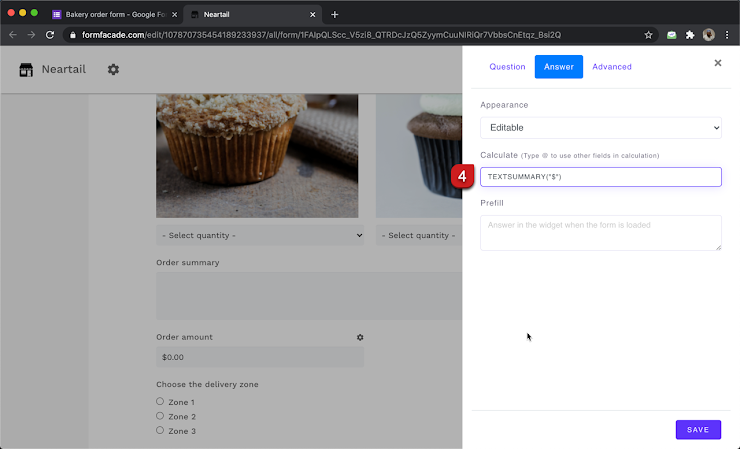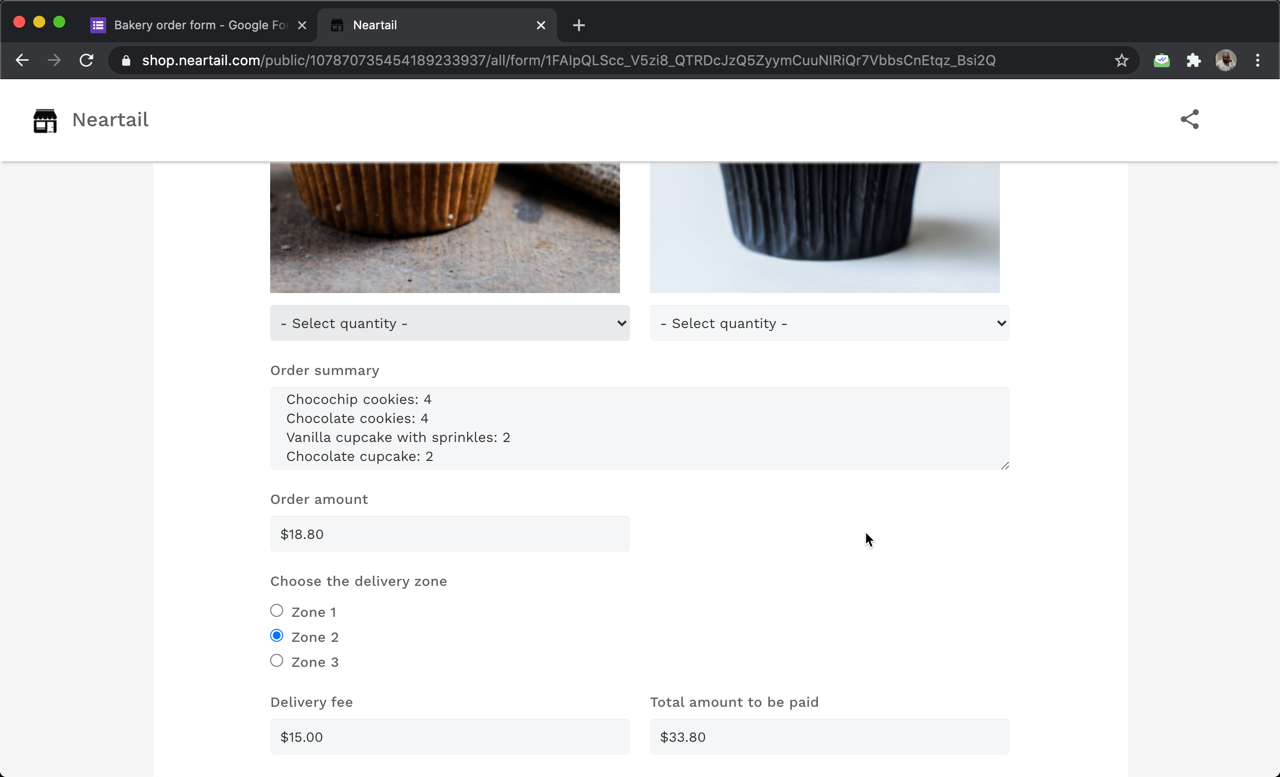### You can use the SUMMARY function to show the list of products selected by the user so that they can review it before placing their order. If you want to record this order summary in Google Forms, you can use the TEXTSUMMARY function.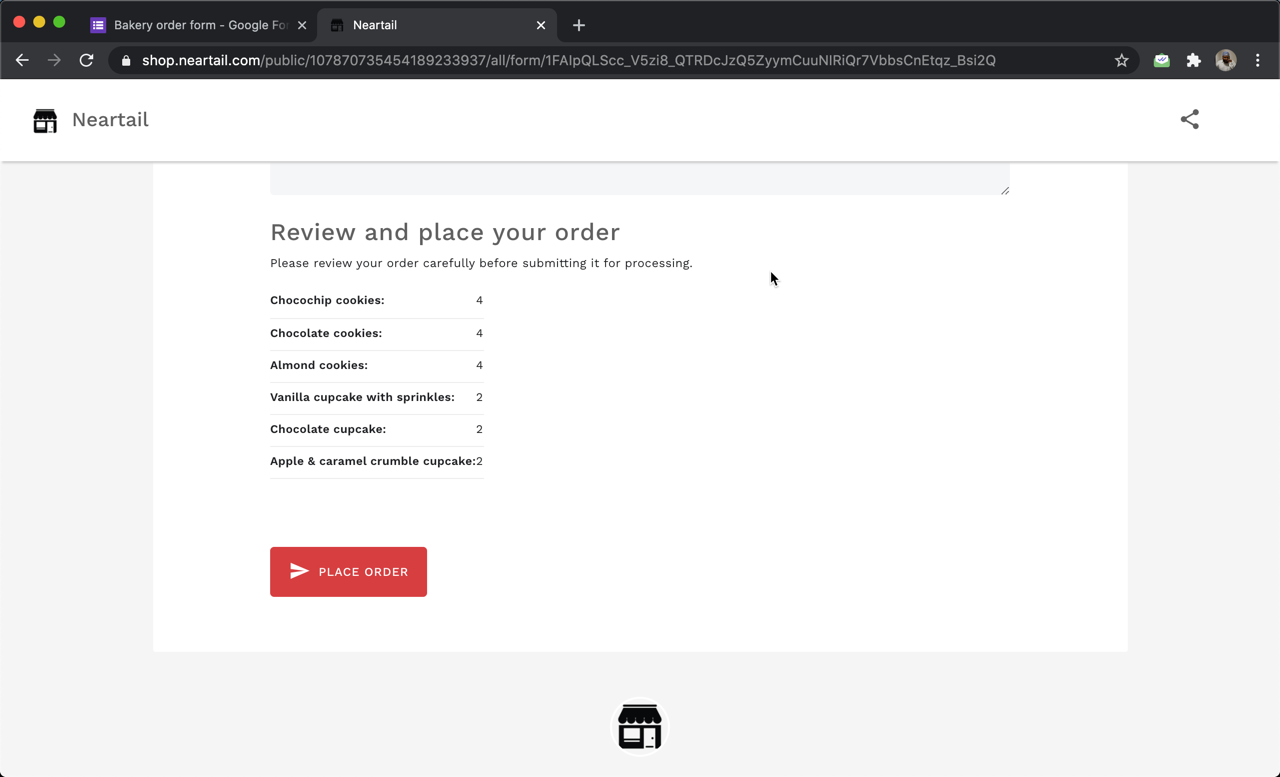#### How to show a summary of the products ordered by the user?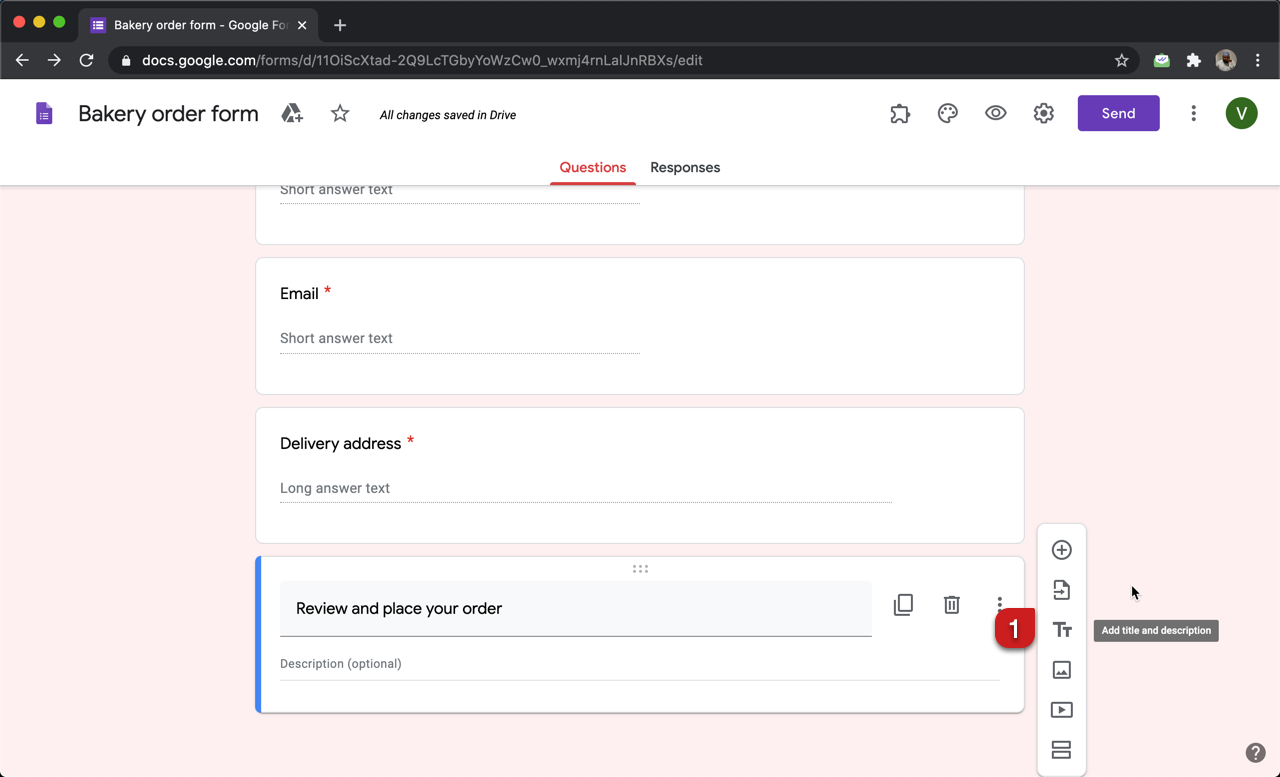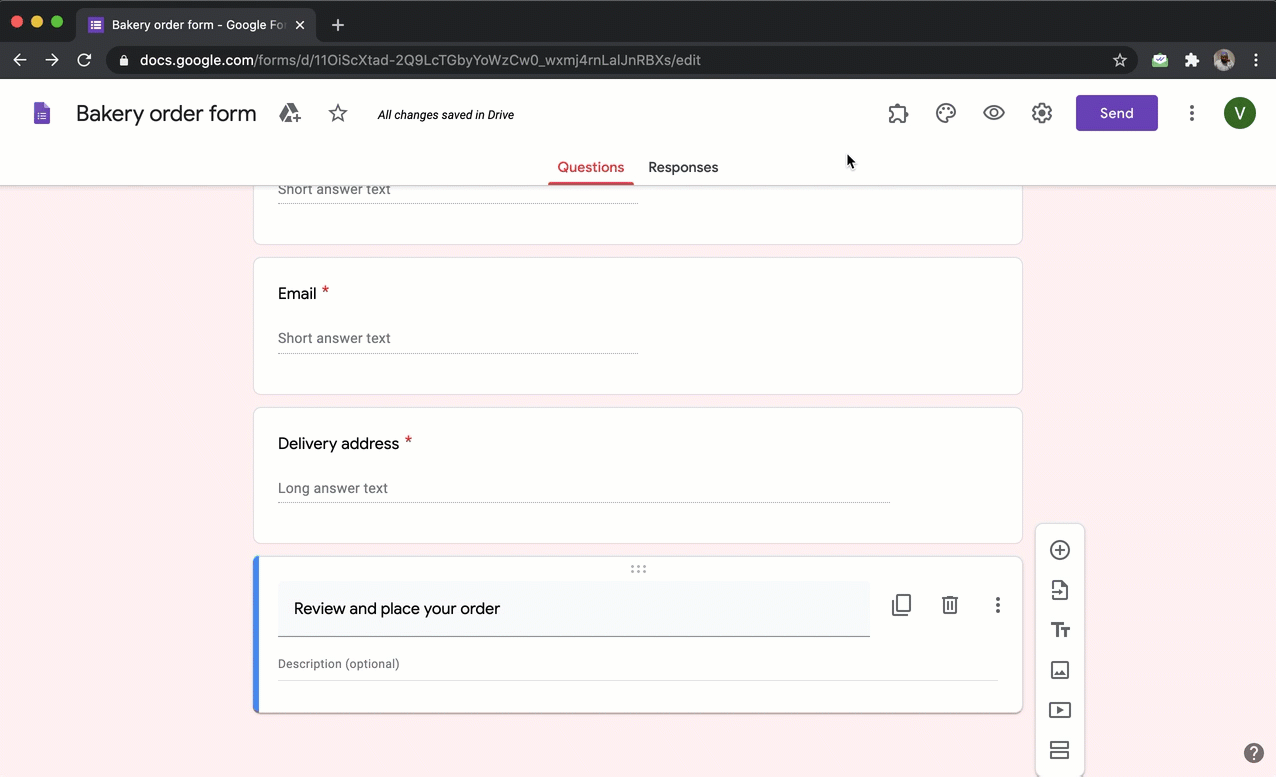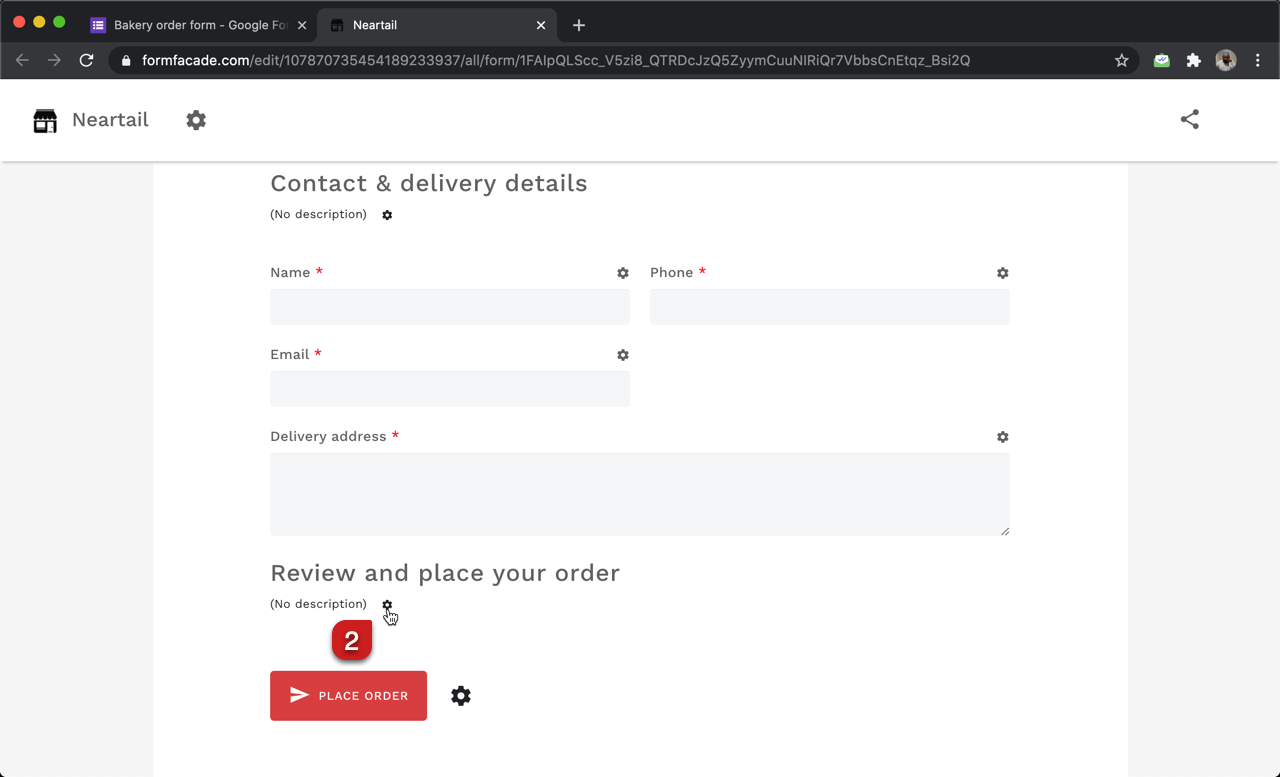#### Use SUMMARY function to show the list of products ordered by the user

You can use the SUMMARY function to show the order summary to the users. The syntax for the SUMMARY function is:

SUMMARY(currency) where

• currency - identifier to find the price in the description/answer choices. The currency can be a symbol or text, but it has to exactly match the format used in the description/answer choices.

Note: The summary function returns a html. So it must be used in the description as a string literal. Any function that you write in the description must be written between \${ ... }

If the currency used in the form is \$, then the summary formula would be \${SUMMARY("\$")}
If the currency used in the form is €, then the summary formula would be \${SUMMARY("€")}
If the currency used in the form is ₹, then the summary formula would be \${SUMMARY("₹")}
Similarly, you can write the summary formula for any currency.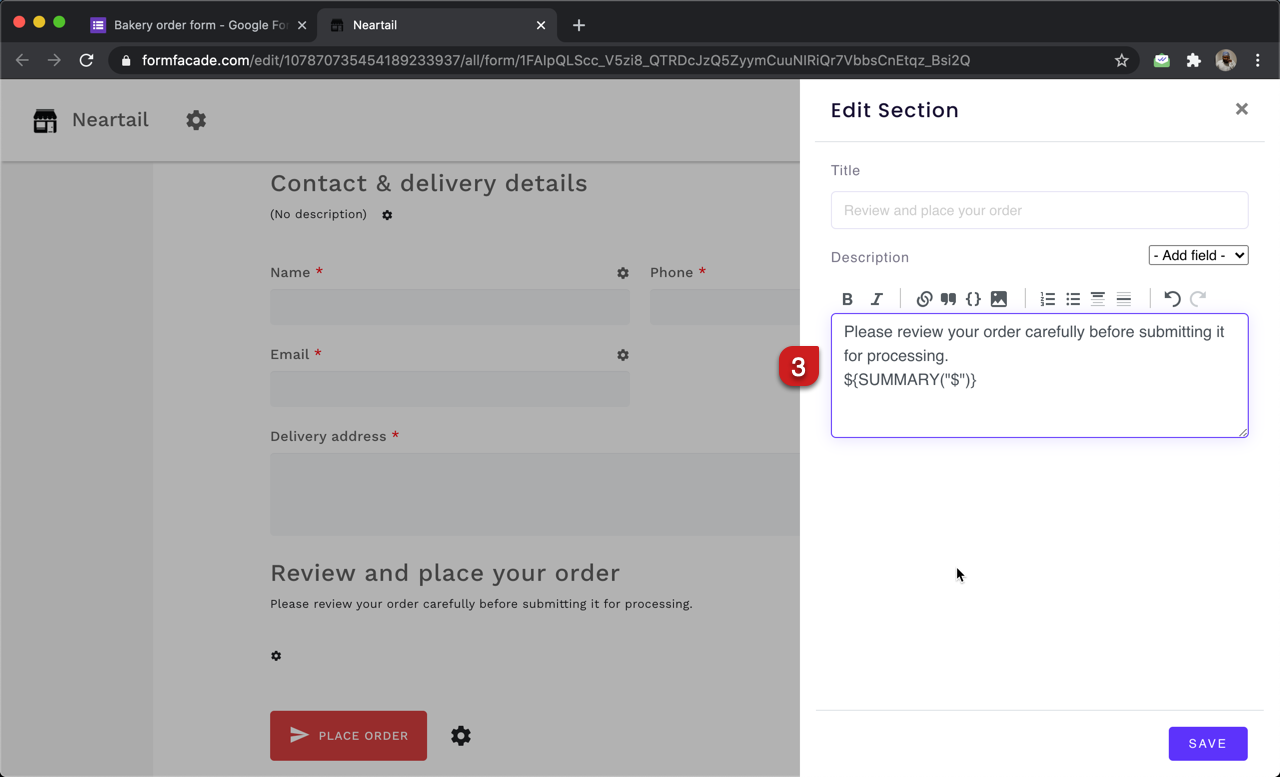#### How to show an order summary and record it in Google Forms?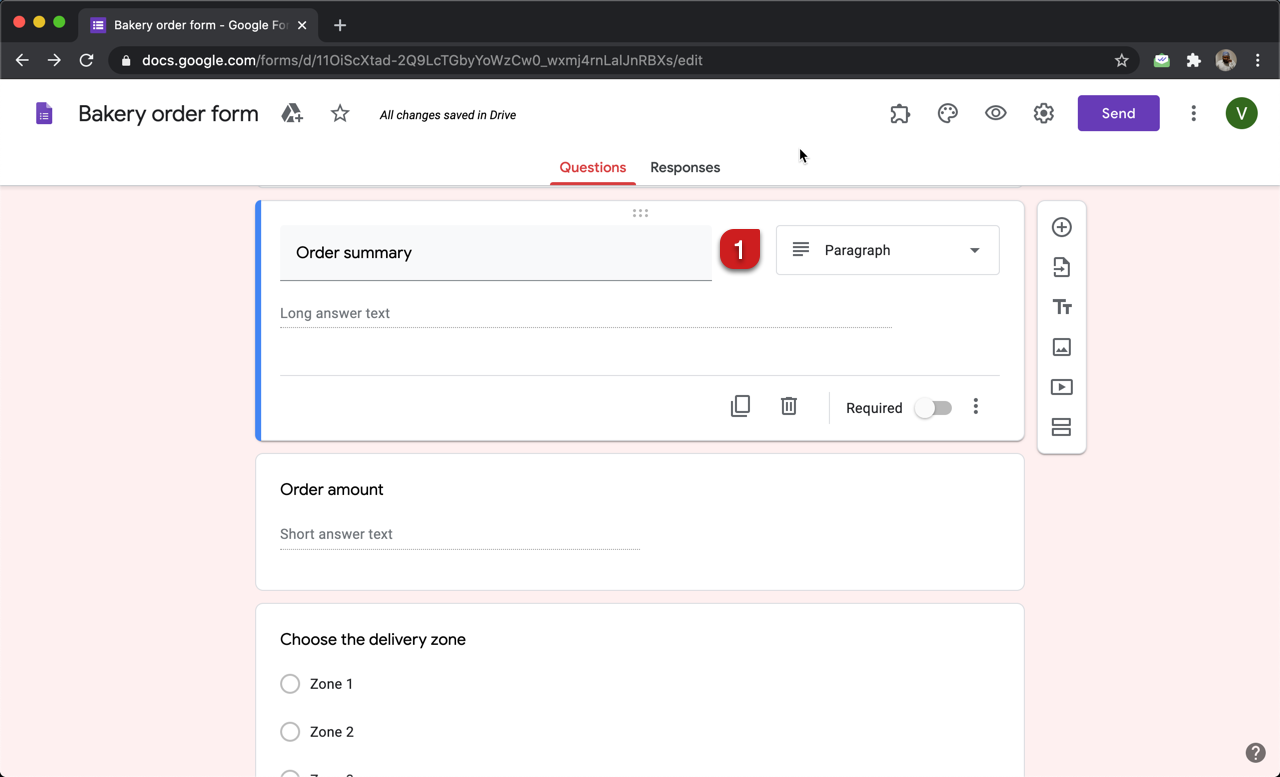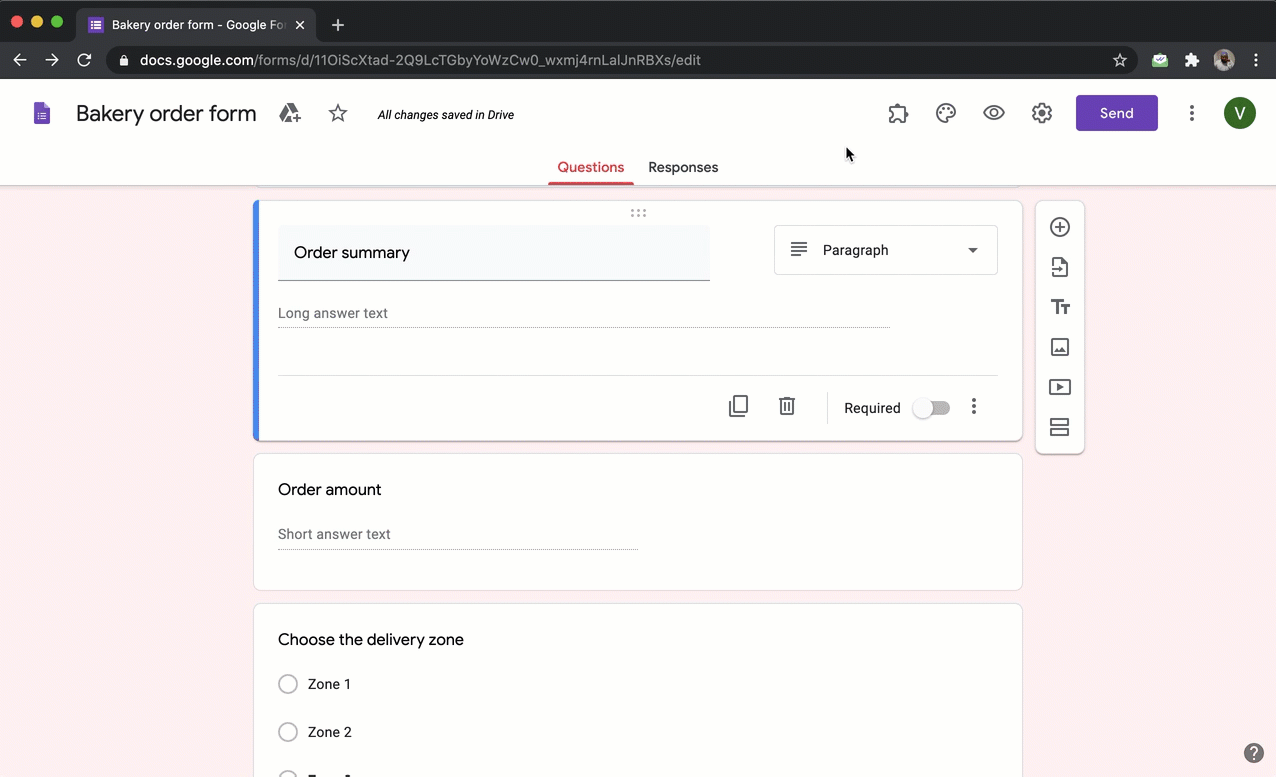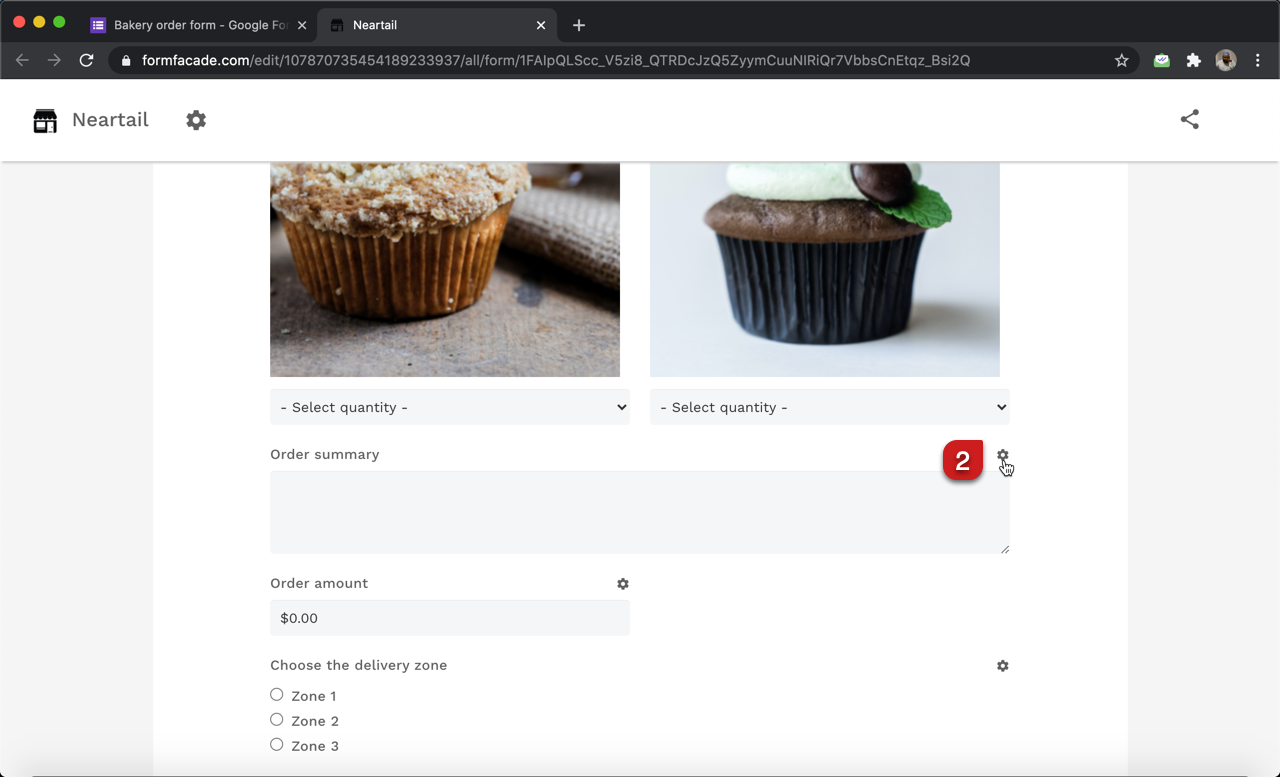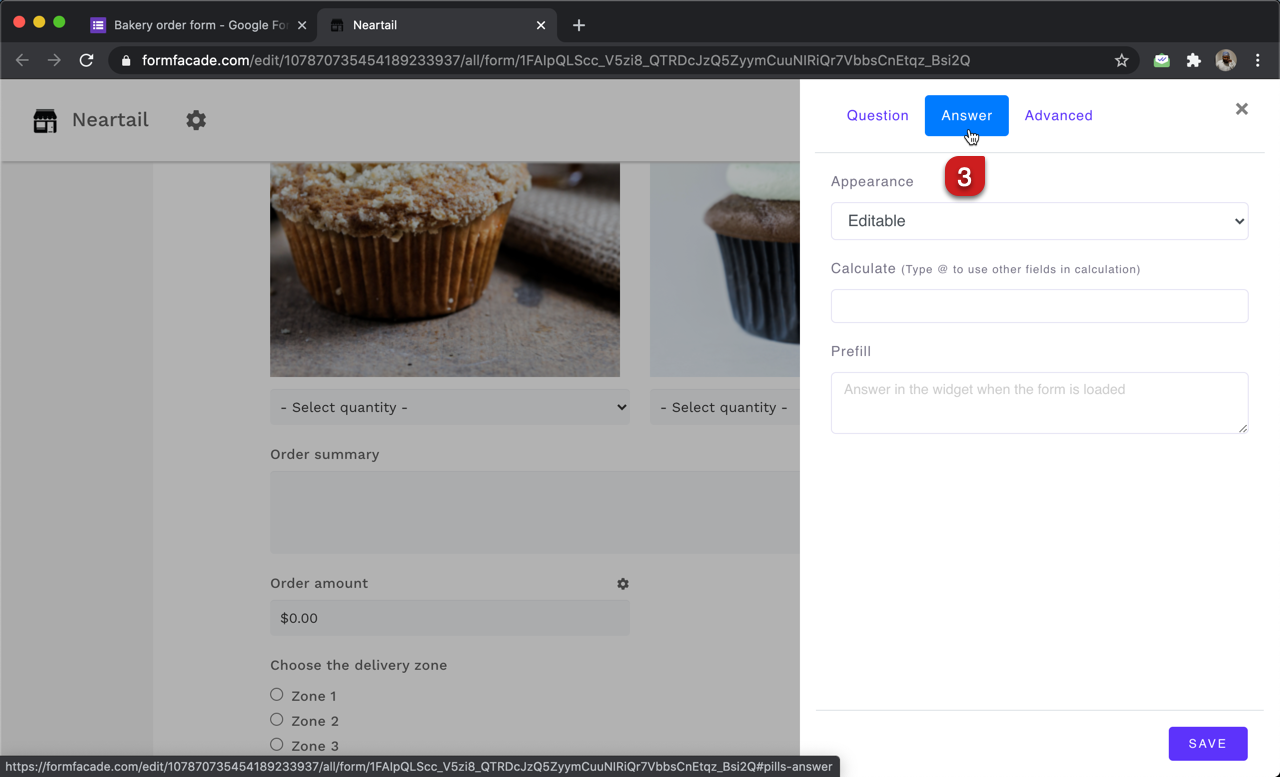#### Use TEXTSUMMARY function to show the order summary and also record it in Google Forms

You can use the TEXTSUMMARY function to show the order summary. Unlike the SUMMARY function, this returns the summary as text. The syntax for the TEXTSUMMARY function is:

TEXTSUMMARY(currency)
where

• currency - identifier to find the price in the description/answer choices. The currency can be a symbol or text, but it has to exactly match the format used in the description/answer choices.

If the currency used in the form is \$, then the summary formula would be TEXTSUMMARY("\$")
If the currency used in the form is €, then the summary formula would beTEXTSUMMARY("€")
If the currency used in the form is ₹, then the summary formula would be TEXTSUMMARY("₹")
Similarly, you can write the summary formula for any currency.